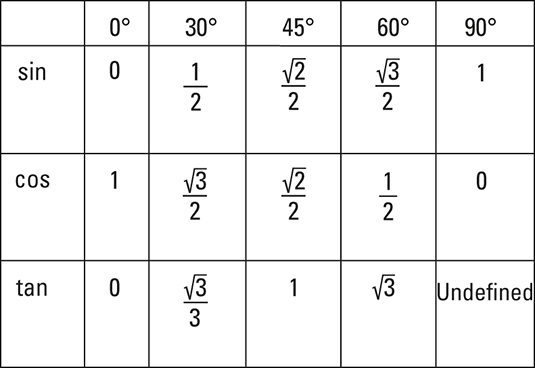##### Trigonometry For Dummies, 3rd Edition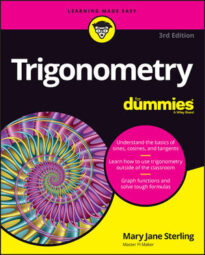Trigonometry is the study of triangles, which contain angles, of course. Get to know some special rules for angles and various other important functions, definitions, and translations. Sines and cosines are two trig functions that factor heavily into any study of trigonometry; they have their own formulas and rules that you’ll want to understand if you plan to study trig for very long.

Many of the formulas used in trigonometry are also found in algebra and analytic geometry. But trigonometry also has some special formulas usually found just in those discussions.

A formula provides you a rule or equation that you can count on to work, every single time. A formula gives a relationship between particular quantities and units. The main trick to using formulas is to know what the different letters represent.

In the formulas given here, you have: r (radius); d (diameter or distance); b (base or measure of a side); h (height); a, b, c (measures of sides); x, y (coordinates on a graph); m (slope); M (midpoint); h, k (horizontal and vertical distances from the center); θ (angle theta); and s (arc length).

The formulas particular to trigonometry have: sin (sine), cos (cosine), and tan (tangent), although only sin is represented here.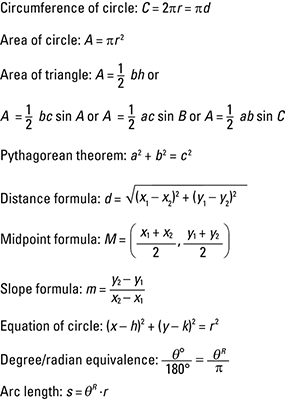## Special right triangles

Every right triangle has the property that the sum of the squares of the two legs is equal to the square of the hypotenuse (the longest side). The Pythagorean theorem is written: a2 + b2 = c2. What’s so special about the two right triangles shown here is that you have an even more special relationship between the measures of the sides — one that goes beyond (but still works with) the Pythagorean theorem.

When you have a 30-60-90 right triangle, the measure of the hypotenuse is always twice the measure of the shortest side, and the other leg is always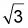or about 1.7 times as big as the shortest side. With the isosceles right triangle, the two legs measure the same, and the hypotenuse is always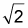or about 1.4 times as long as those two legs.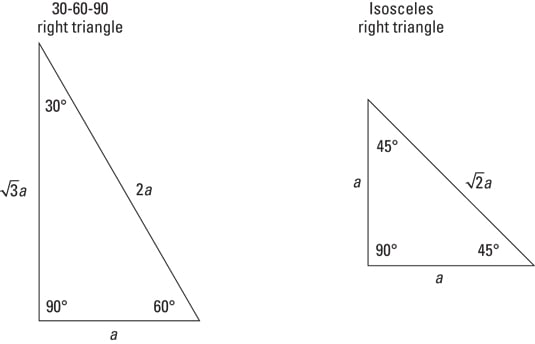## Right triangle definitions for trigonometry functions

The basic trig functions can be defined with ratios created by dividing the lengths of the sides of a right triangle in a specific order. The label hypotenuse always remains the same — it’s the longest side. But the designations of opposite and adjacent can change — depending on which angle you’re referring to at the time. The opposite side is always that side that doesn’t help make up the angle, and the adjacent side is always one of the sides of the angle.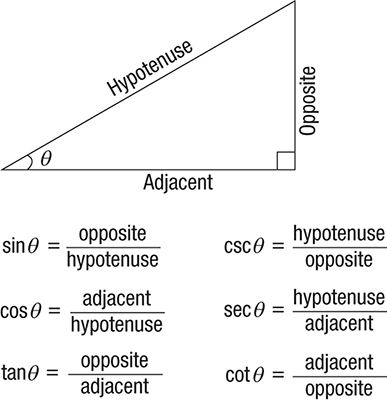## Coordinate definitions for trigonometry functions

The trig functions can be defined using the measures of the sides of a right triangle. But they also have very useful definitions using the coordinates of points on a graph.

First, let let the vertex of an angle be at the origin — the point (0,0) — and let the initial side of that angle lie along the positive x-axis and the terminal side be a rotation in a counterclockwise motion. Then, when the point (x,y) lies on a circle that’s intersected by that terminal side, the trig functions are defined with the following ratios, where r is the radius of the circle.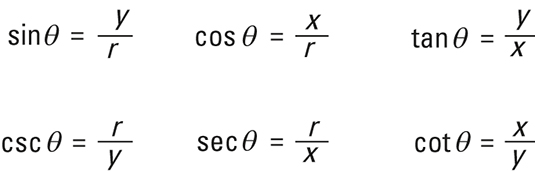## Signs of trigonometry functions in quadrants

An angle is in standard position when its vertex is at the origin, its initial side is on the positive x-axis, and the terminal side rotates counterclockwise from the initial side. The position of the terminal side determines the sign of the various trig functions of that angle. The following shows you which functions are positive — and you can assume that the other functions are negative in that quadrant.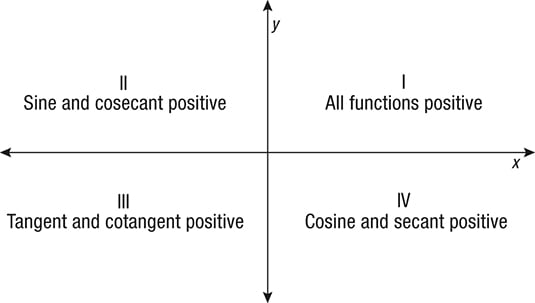## Degree/radian equivalences for selected angles

As you study trigonometry, you’ll find occasions when you need to change degrees to radians, or vice versa. A formula for changing from degrees to radians or radians to degrees is: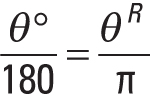The formula works for any angle, but the most commonly used angles and their equivalences are shown below.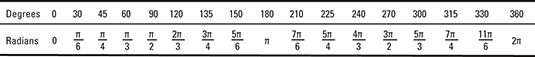## Laws of sines and cosines

The laws of sines and cosines give you relationships between the lengths of the sides and the trig functions of the angles. These laws are used when you don’t have a right triangle — they work in any triangle. You determine which law to use based on what information you have. In general, the side a lies opposite angle A, the side b is opposite angle B, and side c is opposite angle C.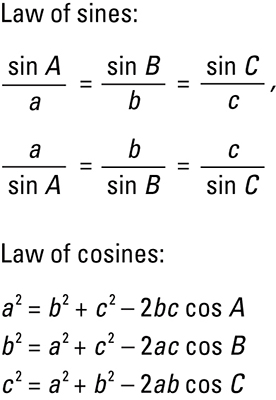## Exact trigonometry functions for selected acute angles

Using the lengths of the sides of the two special right triangles — the 30-60-90 right triangle and the 45-45-90 right triangle — the following exact values for trig functions are found.

Using these values in conjunction with reference angles and signs of the functions in the different quadrants, you can determine the exact values of the multiples of these angles.